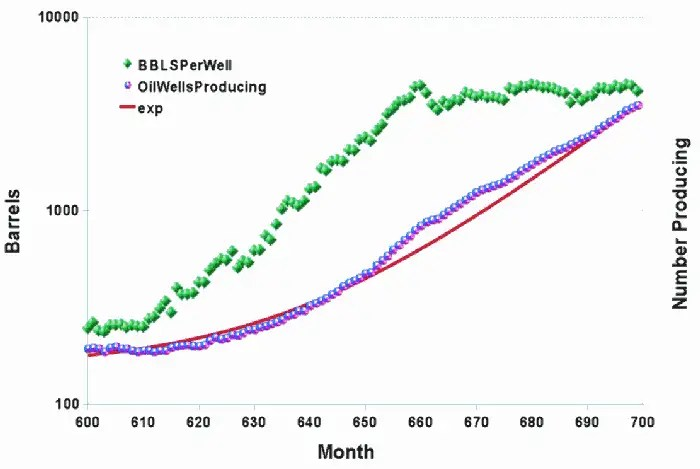# Bakken Growth

Here is the cornucopian mystery of the Bakken shale revealed. When one looks at the production of the Bakken fields, the growth seems remarkable (plotted against data from the North Dakota Dept.of Mineral Resources).Fig 1: Bakken growth, see Fig. 3 for historical

The number of wells producing is growing at an exponential rate while the amount of oil per well is remaining high. How does that work? The total amount produced during a boom period is the convolution of the well number growth rate, N(t), and the depletion rate per well, p(t). The first term is exponentially increasing and the decline rate is exponentially decreasing, see the following chart for the latter.

Assume that all the wells are the average decline rate for the sake of condensing the argument. $$P_T(t) = \int_0^t N(\tau) p(t-\tau) d\tau \\\\ N(t) = e^{a t} \\\\ p(t) = e^{-d t} \\\\ P_T(t) = \frac{e^{a t} – e^{-d t}}{a+d}$$ If the growth is exponential with a greater than zero, then that term will easily dominate over the declining term. Divide P(t) by N(t) and you can see that we have reached the plateau of production proportional to 1/(a+d), which you can see in the figure above. That is full bore acceleration in the number of wells coming on-line. If the growth slows to zero, then the decline kicks in and the cumulative total will start to saturate. So don’t be fooled by exponential growth in well production, since as soon as the construction rate starts to slow, all of those Bakken wells will start to decline and the underlying dynamics will begin to reveal itself. That is the math story behind Gold Rush dynamics and the boom-bust cycle. Everyone gets excited because they can’t see the underlying behavior.

There has been continuous extraction on the Bakken going back 50+ years to 1953.  But only with the recent boom, with hydraulic fracturing techniques, are we seeing the explosion in number of wells. The prior wells were all in serious decline I believe.

This is my plea: What we need from the Dept. of Mineral Resources of NoDak.gov is a complete set of data from every well, not the rolled up info. Even with the rolled up info, you can do some decent analysis, but why not get everything?Fig 3 : Historical Bakken growth, first well to the left was in 1953.

For an exponential and hyperbolic decline analysis on this per county data set and note they are fairly similar in decline.

• McKenzie has a half-life of 0.75 years
• Williams is 1.2 years
• Mountrail is 2.3 years
• Dunn is 18.5 years

If they are hyperbolic declines, the total will be bigger. Dunn seems to be the only set that has any kind of sustain.

 Summary of BakkenInfo Since 1/1/2007 Incremental BOPD Wells Recovery Rec/Well Well Age Curr Ave months BOPD Mountrail 154,089 1,087 154,727,270 142,343 22 141.8 McKenzie 96,750 533 45,059,945 84,540 9 181.5 Dunn 75,560 613 52,446,391 85,557 22 123.3 Williams 69,513 472 30,550,213 64,725 10 147.3 Major Cos 395,911 2,705 282,783,819 Total 483,706 3,187.00 327,866,294 102,876.15 17.00 141.50

Here is a link to an Wolfram Alpha solver for exponential decline, the numbers are taken from the table above for Williams county. (The number 30 is the average days in a month, as the calculation assumes a daily production rate)Fig. 4 : Wolfram Alpha algorithm for predicting decline rate from Rec/Well=64725, WellAge=10,CurrAveBOPD=147.3

For hyperbolic decline, example using McKenzie, rates are in per day.